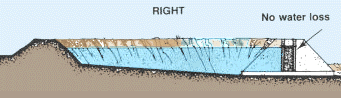# 2. ESTIMATES OF WATER REQUIREMENTS

## 2.0 Water to fill the pond

To determine how much water your pond will hold when it is full you need to calculate:

### How to calculate the surface area of the pond

If the pond has a square shape, multiply two sides (in metres, or m) or, if it has a rectangular shape, multiply the length (in m) by the width (in m) to find the surface area (in square metres or m2).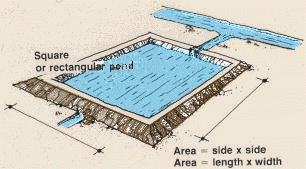Examples

If you have a large pond you may want to convert the surface area from square metres to ares or hectares (ha).

100 m2 = 1 are, 10000 m2 = 100 ares = 1 hectare (ha)

Examples

 If the pond has an irregular shape but the sides are generally straight, you can find the surface area by dividing the pond into smaller areas that can be more easily calculated, and add these to find the total surface area. Prepare a plan of the surface area of the pond, as accurately as possible, on a sheet of paper. Now divide the plan into squares, rectangles, right (or 90°) triangles.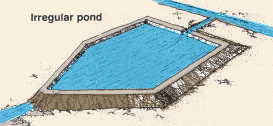Note: when dividing the surface of a large irregular pond, it may help to trace an xy axis the length of the plan. You can use this axis as reference line along which you can construct squares, rectangles or triangles.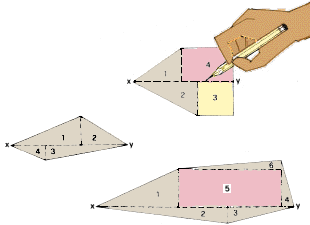Calculate the area of each square, rectangle or triangle using accurate length, width, base and height measurements (in m). To find the area of a square, multiply two sides; To find the area of a rectangle, multiply the length by the width; To find the area of a right (or 90°) triangle, multiply the base by the height and divide by 2 After you have calculated all of the smaller areas, add them to find the total surface area. If the pond has an irregular shape with a curving side, you may need to approximate the curved part to find the surface area. Construct a line across the curved side of the pond so that the part outside the line is approximately the same as the part inside. Then calculate area or areas as you did earlier in this section.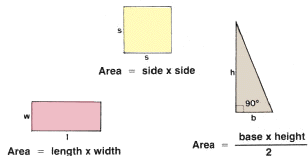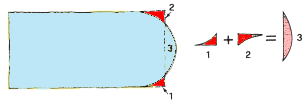Examples The parts outside the pond and the part inside the pond are about equal; assume 1+2 = 3: you can calculate the surface area by multiplying the length by the width.

 The parts outside the pond and the part inside the pond are about equal;assume 2+3 = 4; the total surface is then = ADE+FCB+EDCF = 1a+1b+1c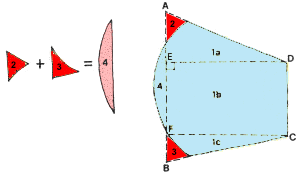### How to calculate the average water depth of the pond when it is empty

If the pond is not too large, you can mark the future water level with strings stretched across the pond and tied to stakes at AB, CD, and EF. The stakes are placed at the planned water level. Measure the depth in a number of places along each string and calculate the average water depth as shown below.

If the pond is large and it will be difficult or impossible to stretch strings from bank to bank, you may be able to calculate the average water depth using a combination of strings where possible, and a square grid, as shown below.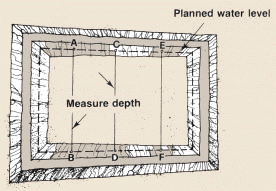### How to calculate the average water depth of the pond when it is full

If the pond is small, with a regular shape, and has a bottom with a constant slope from one end to the other, go into the water and measure the depth at four points, 1, 2, 3 and 4 in the pond. To find the average depth, calculate the average of these measurements.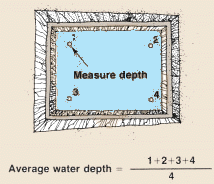If the pond is large, with a regular shape, and has a bottom with a constant slope from one end to the other, increase the number of measurements. Go into the water and measure the depth at nine or more points in the pond.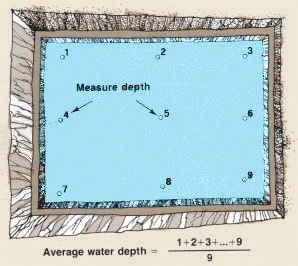If the pond is large with an irregular shape and an irregular bottom, construct a square grid 5 m x 5 m over the pond surface. Go into the water and measure the depth at each grid intersection. Average all measurements.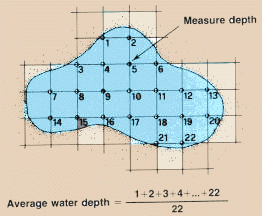### How to calculate the volume of water in the pond

You have thus calculated the surface area of the pond and the average water depth of the pond. Now, using the figures you have found, you can calculate the volume of water in the pond by multiplying the surface in square metres (m2) by the average water depth in metres (m) to get the volume of the pond in cubic metres (m3).

SURFACE AREA x AVERAGE DEPTH = VOLUME

Examples

 Surface area (m2) Average water depth (m) Water volume (m3) 235 x 1.0 = 235 450 x 1.2 = 540 2500 x 1.5 = 3750

 Note: 1 cubic metre (m3) = 1000 litres (l). To express water volume (in m3) in litres (l) multiply by 1000. To express water volume (in l) in cubic metres (m3) divide by 1000.## 2.1 Water losses by seepage

Water that is lost vertically through the bottom of the pond, horizontally through the dikes by infiltration, and through the drainage system of the pond is called seepage water.

If the dikes of your pond are well built and well maintained and if the drainage system is watertight, the amount of seepage water lost horizontally will be very small. You will need to calculate only the vertical seepage.

Water seepage will be greater from a new pond when it is filled for the first time. The soil structure of the pond will still be good and water will be lost.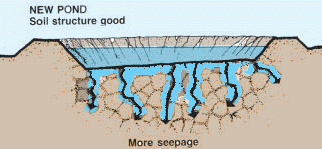After the pond has been filled with water for some time, the water tends to break down the soil structure and the soil pores become sealed by organic matter that collects on the pond bottom. As a result, the soil permeability and losses by seepage will decrease.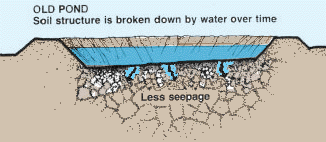The amount of vertical water seepage will depend on the soil texture and on the soil structure of the pond bottom. If the composition of the soil is coarse, as in sandy soils, it will be permeable, and water will be lost by seepage. Soils with a good structure will allow more seepage than soils with a bad structure.

### How to calculate water losses caused by seepage

The figures below give the rate of seepage losses in millimetres per day (mm/day) from various soil types (in the natural state) needed to calculate pond seepage losses over a period of time.

 Natural soil type Seepage losses (mm/day) Sand 25.00 - 250 Sandy loam 13.00 - 76 Loam 8.00 - 20 Clayey loam 2.50 - 15 Loamy clay 0.25 - 5 Clay 1.25 - 10

Example

### Reducing seepage water losses by puddling

One way to reduce seepage water losses is to break the soil structure of the pond bottom before it is filled with water. This is a common practice in irrigated rice fields, and is called puddling.

The soil of the pond bottom is first saturated with water. The amount of water you will need initially to saturate the bottom (200-300 mm) will vary slightly with the type of soil. Assume a standard requirement of 300 mm, or 0.3 m.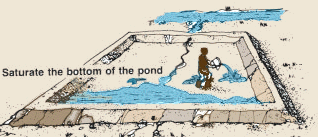When the water has soaked into the soil of the pond bottom enough to permit working, you are ready to puddle. This is done by hoeing, ploughing or working the soil by any other suitable means.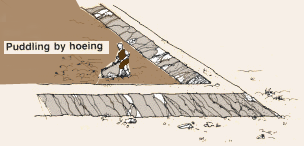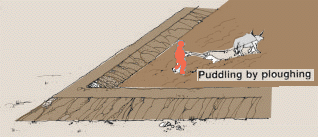### How to calculate water needed for puddling and water losses by seepage after puddling

To calculate the amount of water needed for puddling multiply the pond area (in m2) by 0.3 m.

Example

The figures in the chart give the rate of seepage losses from various soil types (after puddling) needed to calculate pond seepage losses over a period of time.

 Puddled soil type Seepage losses (mm/day) Sandy loam 3-6 Loam 2-3 Clayey loam 1-2 Loamy clay about 1 Clay about 1

Example

 To calculate the total water required both for puddling and to compensate for seepage losses for 6 months thereafter, add the two values. Example Water required for puddling as calculated in the example above: 450 m3. Water losses by seepage for 6 months (from previous example): 810 m3. Total water needed: 450 m3+ 810 m3 = 1 260 m3.

## 2.2 Water losses by evaporation

The water that is lost to the air from the surface of the pond is called evaporation. The amount of water lost by evaporation depends largely on local climate conditions.

High air temperatures, low humidity, strong winds and sunshine will increase evaporation.

Low air temperatures, high humidity, rainfall and cloud cover will decrease evaporation.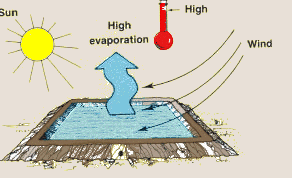Evaporation will also depend on the water surface area. The larger the pond, the more water will evaporate from its surface.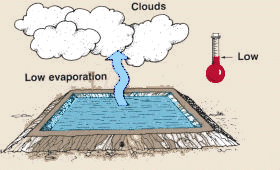### Evaporation rates

You will need to know your local evaporation rate in order to calculate the amount of water lost from the surface of a pond by evaporation. Evaporation rates, which are provided by meteorological stations, are found by measuring and recording water losses by evaporation over many years.

Evaporation rates are usually expressed as the water depth lost in millimetres over a period of time, e.g., 2 mm/day, 14 mm/week or 60 mm/month.

### Evaporation rates by Class A Pan

One of the most common methods to find the evaporation rate is accurately to measure daily water losses from a standard-size container called a Class A Pan. Evaporation rates by Class A Pan can be obtained from many meteorological stations throughout the world.

In choosing a meteorological station for evaporation rates, be careful to select one where climatic conditions such as sun, wind and rainfall are similar to conditions in your locality. If you are not sure ask the advice of a technician from the meteorological station.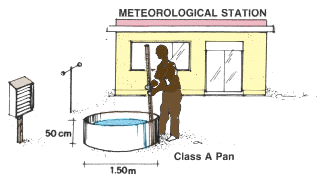Class A Pan evaporation rates may be expressed as either mm/ day, mm/week or mm/month, over a period of years. Usually you will be able to obtain the average monthly evaporation rates, which are based on measurements made during several years. If you can get average monthly evaporation rates, this will be the most convenient for calculating water losses by evaporation.

Note: water evaporates faster from a Class A Pan than from a large water surface such as a pond. When using Class A Pan evaporation rates you must multiply by a correction factor of 0.75 to better approximate evaporation losses.

Example

### How to calculate water losses by evaporation using Class A Pan evaporation rates

To calculate evaporation losses, multiply the water surface area (in m2 ) by the corrected evaporation rate (in m) for the length of time your pond will be in use.

• Obtain Class A Pan average evaporation rates (in mm) for each month your pond will be full from an appropriate meteorological station;
• Class A Pan average monthly evaporation rates needed for this example are as shown below:
 Month Evaporation rate (mm) April 56 May 63 June 68 July 75 August 84 September 79
• Add the rates (in mm) for each month and multiply this sum by 0.75 (correction factor for Class A Pan rates) to find the total corrected evaporation (in mm) for all the months;
• Divide this total corrected evaporation (in mm) by 1 000 to express the evaporation in metres;
• Multiply this value (in m) by the water surface area (in m2) to find the total water losses by evaporation (in m3) for the months your pond will be in use.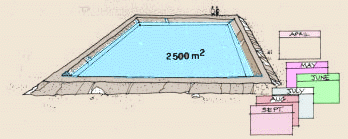Example

### Evaporation rates by the Penman Formula

Some meteorological stations may not record evaporation rates using a Class A Pan. If this is the case, you may be able to get evaporation rates calculated by the Penman Formula. The Penman Formula is based on data of atmospheric pressure, radiation, sunshine, humidity, air temperature and wind speed.

Note: under some conditions, such as when there are high winds, and especially in arid climates, the Penman Formula may provide evaporation rates that are too low. If this is the case in your locality, ask the advice of a technician from the meteorological station.

The evaporation rates calculated by the Penman Formula are more accurate than the rates recorded using a Class A Pan. To calculate evaporation losses by Penman rates, you can use the method shown above but, since these rates are more accurate, omit the step where you multiply the total evaporation by the correction factor of 0.75.

## 2.3 Total water requirements

The total water requirements for a pond are:

• The amount of water needed to fill the pond in a reasonable length of time;
• The amount of water needed to compensate for seepage and evaporation losses over the planned fish-growing period.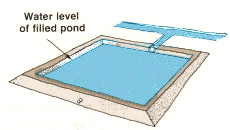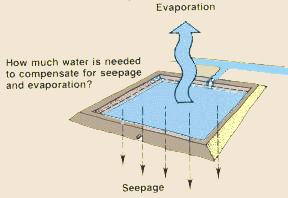### Pond size and water flow required

To begin growing fish as soon as possible, you should have enough water available to fill your pond in a reasonable length of time. For ponds smaller than 1 500 m3 , eight days is reasonable.

Before you begin to build a pond it will be helpful to compare the number of days needed to fill ponds of various sizes and the rate of water flow required. Table 1 will give you a quick idea of some of the combinations possible.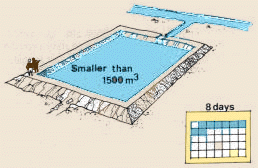Approximate filling time (days) Pond volume (m3) Required water flow (l/s) 8 400 0.5 1000 1.5 2500 3.5 10000 14.0 4 400 1.0 1000 3.0 2500 7.0 5000 14.0 10000 28.0 2 400 2.0 1000 6.0 2500 14.0 10000 56.0

Examples

If you measure the available water flow (see Section 3) before you begin to build your pond, you will be able to estimate more precisely the number of days needed to fill a pond. Table 2 gives the water volume per day (in m3) provided by various rates of water flow. To calculate the number of days to fill a pond, divide the planned pond water volume by this daily water flow.

TABLE 2

Amount of water provided per day by various rates of water flow

 l/s l/min l/h l/day m3/day 1 60 3600 86400 86.4 2 120 7200 172800 172.8 3 180 10800 259200 259.2 4 240 14400 345600 345.6 5 300 18000 432000 432.0 6 360 21600 518400 518.4 7 420 25200 604800 604.8 8 480 28800 691200 691.2 9 540 32400 777600 777.6 10 600 36000 864000 864.0 14 840 50400 1209600 1209.6 15 900 54000 1296000 1296.0 20 1200 72000 1728000 1728.0 1Z Zx60 Zx3600 Zx86400 Zx86.4

1 The bottom line of this table shows how to convert water flow values (Z) in l/s into l/min, l/h, l/day and m3 /day.

Example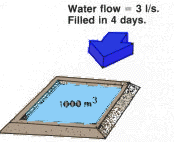Using Table 2, you find that a water flow of 3 l/s will provide 259.2 m3 of water per day.

The time required to fill your pond is 1000 m3 ÷ 259.2 m3 /day = 3.86 days, say 4 days.

As a check, compare this result with Table 1 and you will confirm this by reading across from 4 days that you need 3 l/s to fill a pond of 1000 m3.

### Pond volume and the number of ponds possible will depend on the water flow available

The size and number of ponds you will be able to build will depend on the water flow available at the time you plan to fill them. The paragraphs above together with Tables 1 and 2 give you several ways to estimate the pond volume possible with various rates of water flow.

Now you must decide on the volume of each pond to be built, on the number of ponds to be built and how you will plan for the future expansion of your fish-farming operations.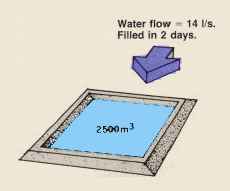### Volume of each pond to be built

You have measured the water flow and have found that you have 14 l/s available:

• Using Table 1 you find that with 14 l/s you can fill one pond of 2500 m3 in 2 days;
• Or, with 14 l/s, you can fill one pond of 5000 m3 in 4 days;
• Using the values in Table 1 you can also calculate that with 14 l/s you can fill one pond of 10 000 m3 in 8 days.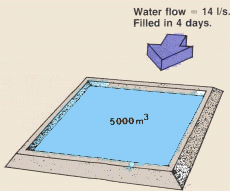### Number of ponds to be built

With the same water flow of 14 l/s, you may decide to build more and smaller ponds than shown above:

• For example, with 14 l/s, you can fill two ponds of 2500 m3 (= 5000 m3) in 4 days;
• Or, with 14 l/s, you can fill five ponds of 500 m3 (= 2500 m3 ) in 2 days.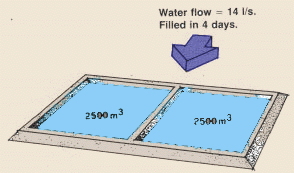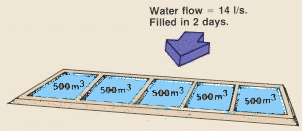### Planning for future expansion

You may also decide to build only one pond this year and another next year:

• With 14 l/s you can build one pond of 2 500 m3 this year and fill it in 2 days and expand your operation next year to two ponds of 2 500 m3 that can both be filled in 4 days with the water flow available.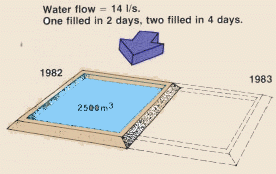Note: when you have several ponds, they need not be filled at the same time. First fill one and then another as your water supply permits.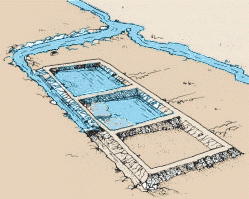### Losses by seepage and evaporation

In addition to the water needed initially to fill a pond, you will need to add water regularly, over the length of the growing season, to compensate for seepage and evaporation losses.Before you begin to build a pond you should estimate how much water you will need to compensate for seepage and evaporation losses, per hectare of pond area, so that your available water supply will be sufficient during the driest season. On this basis, you can then calculate the pond area that can be maintained with this minimum water flow only.

Remember:
1 ha = 10 000 m2
1 m3 = 1 000 l
1 day = 86 400 s

Example

 Note: when adding water to a pond to compensate for seepage and evaporation losses, add only enough water to keep the water level at the normal height. If you add too much water, the rich, fertilized pond water that feeds the fish will be washed away through the outlet. Check the outlet regularly to see that it is watertight and that no water is being lost.